### Another try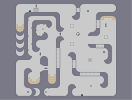Hover over the thumbnail for a full-size version.

Author TRT_Bardock action agd author:trt_bardock playable unrated 2009-05-21 2009-05-21 4 more votes required for a rating. \$Another try#TRT_Bardock#action#1111111111111111111111111111111111111111111111111111111111111111111119000006<6<6<619000000060000007=7=7=7100000000000;1111111111100;111<000019000000006=00:9061870010;111111<000000001:=00101906196=000000071870010100710000;1@?1111110010180:=0000:1PN1111960010:=000000000000061000018000000000000000710000:111<0;11000001111=00000006=0:9000000000000000000000000000000000007000000000000;8000000;11807<00000000:1001<00:96101=000000000000610000090000000000000000000000000000000000000000000000000000;00<0000000000000000007100100000000000000;1001=00000000000000000190000000000000187<0000100000001807<00:11=000010000000:111=0000000087180000000000000000007111111111111111111111111111111111111111111111111111111111111111111111|5^636,150!0^294,450!0^294,462!0^294,474!0^294,486!0^330,450!0^330,462!0^330,474!0^330,486!0^306,462!0^318,462!0^318,474!0^306,474!0^306,486!0^318,486!0^306,498!0^318,498!0^330,498!0^294,498!10^528,66!4^492,570,1!7^588,444,3!7^564,444,3!7^540,444,3!7^516,444,3!7^516,444,1!7^540,444,1!7^564,444,1!7^588,444,1!7^492,444,3!7^492,444,1!10^624,312!7^612,276,3!7^612,276,1!7^588,276,3!7^588,276,1!7^588,132,2!7^588,156,2!7^588,156,0!7^444,372,2!7^444,372,0!7^444,396,2!7^444,396,0!0^198,492!0^222,492!0^234,492!0^222,504!0^210,504!1^540,360!7^348,348,2!7^348,348,0!7^348,372,2!7^348,372,0!7^348,396,2!7^348,396,0!0^210,492!0^198,504!0^186,504!0^186,492!0^174,492!0^174,480!0^234,480!0^234,468!0^174,468!0^186,480!0^222,480!7^348,300,2!7^348,300,0!7^348,324,2!7^348,324,0!7^468,60,2!7^468,60,0!6^468,204,5,0,1,NaN!0^138,174!0^138,162!0^138,150!0^102,150!0^102,162!0^102,174!0^114,162!0^126,162!0^126,174!0^114,174!0^114,186!0^126,186!0^102,186!0^138,186!12^168,120!9^276,552,0,0,10,13,1,-1,0!12^294,576!12^294,528!12^264,144!12^234,216!12^216,162!12^192,168!12^168,216!12^192,264!12^168,300!9^258,174,0,1,18,8,0,0,0!9^258,174,0,1,10,7,0,-1,0!12^216,180!12^234,144!1^432,264!12^282,156!12^259,206!12^217,202!9^168,552,1,0,11,17,1,0,-1!1^432,156!0^630,102!0^618,102!0^642,102!0^606,102!0^606,114!7^588,132,0!0^654,102!0^666,102!0^678,102!0^690,102!0^690,114!0^618,114!0^678,114!1^636,222!4^180,66,1!11^216,60,168,552!9^546,516,0,0,15,8,1,-1,0!9^540,360,0,0,9,1,1,-1,0# at NUMA...

## Other maps by this author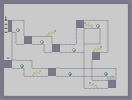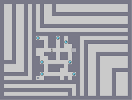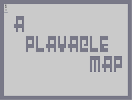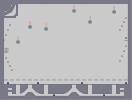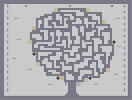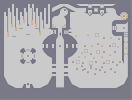Twisted Origins DronePaths_Level_1 A Playable Map Falling Thwumplets Rotten apples It's all in the head